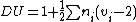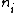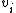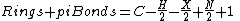xDegree of unsaturationEncyclopedia
The degree of unsaturation (also known as the index of hydrogen deficiency (IHD) or rings plus double bonds) formula is used in organic chemistry
Organic chemistry
Organic chemistry is a subdiscipline within chemistry involving the scientific study of the structure, properties, composition, reactions, and preparation of carbon-based compounds, hydrocarbons, and their derivatives...

to help draw chemical structures. The formula lets the user determine how many rings, double bonds, and triple bonds are present in the compound to be drawn. It does not give the exact number of rings or double or triple bonds, but rather the sum of the number of rings and double bonds plus twice the number of triple bonds. The final structure is verified with use of NMR
NMR
NMR may refer to:Applications of Nuclear Magnetic Resonance:* Nuclear magnetic resonance* NMR spectroscopy* Solid-state nuclear magnetic resonance* Protein nuclear magnetic resonance spectroscopy* Proton NMR* Carbon-13 NMR...

, mass spectrometry
Mass spectrometry
Mass spectrometry is an analytical technique that measures the mass-to-charge ratio of charged particles.It is used for determining masses of particles, for determining the elemental composition of a sample or molecule, and for elucidating the chemical structures of molecules, such as peptides and...

and IR spectroscopy, as well as inspection.

## General formula

The formula for degree of unsaturation iswhereis the number of atoms with valence
Valence (chemistry)
In chemistry, valence, also known as valency or valence number, is a measure of the number of bonds formed by an atom of a given element. "Valence" can be defined as the number of valence bonds....

That is, an atom that has a valence of x contributes a total of x-2 to the degree of unsaturation. The result is then halved and increased by 1.

## Rings plus pi bonds formulation

For molecules containing only carbon, hydrogen, monovalent halogens, nitrogen, and oxygen, the formulawhere C = number of carbons, H = number of hydrogens, X= number of halogens and N = number of nitrogens, gives an equivalent result. Oxygen and other divalent atoms do not contribute to the degree of unsaturation, as (2-2) = 0.

The degree of unsaturation is used to calculate the number of rings and pi bond
Pi bond
In chemistry, pi bonds are covalent chemical bonds where two lobes of one involved atomic orbital overlap two lobes of the other involved atomic orbital...

s, where
• Rings count as one degree of unsaturation
• Double bonds count as one degree of unsaturation
• Triple bonds count as two degrees of unsaturation